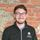cancel
Showing results for
Did you mean:Helper I

## Find a rolling sum for a specific moment in time

Hello,

I am working to find a calculation of our company’s active monthly recurring revenue (MRR) over time. I have raw transaction data that looks like the following. We have the name of the company, the date that the invoice was created, the day their subscription starts and ends, and the actual amount of MRR.What I am looking to do is to find a sum of all the active MRR values for a specific moment in time. MRR would be considered active if the End Date of the transaction is greater than or equal to today (or the day being looked at) to signinify it hasn't ended, and the Start Date is less than or equal to today (or the day being looked at) to signifiy that it has started.

If we were using a date table to look at the current MRR for certain dates, the MRR for the date of 06/23/2021 would be 9. I am looking to create a formula or some way to measure this within a dates table for various moments in time. So if I wanted to find the MRR for 03/16/2019, it would be 15 because Transaction 6 would be live and Transaction 7 would have just started the day prior.

A results Table would look like this:Any advice on how to achieve this would be greatly appreciated! I am guessing I will have to create a dates table that looks at each data and measures based on the filters addressed above. I am just not sure how to properly do this.

1 ACCEPTED SOLUTIONSolution Sage
``````// Create a Date table the way it's
// described in, for instance,
// and keep it disconnected from the
// fact table. If you want to connect
// it to the fact table, all relationships
// should be inactive by default.
//
// ATTENTION: The measure will return the
// total MRR as calculated on the day
// that's the last one visible in the
// current context.
//
// Then create this measure:

[Total MRR] =
var LastVisibleDate = MAX( Dates[Date] )
var Result =
SUMX(
FILTER(
T, // this is the name of your fact
T[Start Date] <= LastVisibleDate
&&
LastVisibleDate <= T[End Date]
),
T[MRR Amount]
)
return
Result``````
2 REPLIES 2Solution Sage
``````// Create a Date table the way it's
// described in, for instance,
// and keep it disconnected from the
// fact table. If you want to connect
// it to the fact table, all relationships
// should be inactive by default.
//
// ATTENTION: The measure will return the
// total MRR as calculated on the day
// that's the last one visible in the
// current context.
//
// Then create this measure:

[Total MRR] =
var LastVisibleDate = MAX( Dates[Date] )
var Result =
SUMX(
FILTER(
T, // this is the name of your fact
T[Start Date] <= LastVisibleDate
&&
LastVisibleDate <= T[End Date]
),
T[MRR Amount]
)
return
Result``````Helper I

Appreciate the help!